Varsity Tutors always has a different Common Core: 5th Grade Math Question of the Day ready at your disposal! If you’re just looking to get a quick review into your busy day, our Common Core: 5th Grade Math Question of the Day is the perfect option. Answer enough of our Common Core: 5th Grade Math Question of the Day problems and you’ll be ready to ace the next test. Check out what today’s Common Core: 5th Grade Math Question of the Day is below.

### Question of the Day: Common Core: 5th Grade Math

Select the equation that reflects the phrase below.

Find the product oftimes the quotient ofdivided by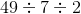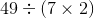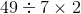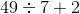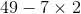The Common Core 5th Grade Math Question of the Day on Varsity Tutors’ Learning Tools can help your child along their path toward mastering the topics in their mathematics curriculum. Each Question of the Day covers a concept that your child is expected to know at their grade level. Fifth graders are required to be proficient in subtracting, multiplying, and dividing fractions with whole numbers and units. They’re also expected to be able to divide using two-digit divisors and use decimals. As your fifth grader continues developing their geometry skills, concepts like volume will be introduced as well.